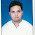# UGC NET Computer Science Paper II June 2010 - Part 1 MCQs

1.       x1 is a clone of x” means x1 is identical to x in terms of the physical attributes namely, height, weight and complexion. Given, height, weight and complexion only form a complete set of attributes for an entity, cloning is an equivalence relation. What is your impression about this statement?
(A) The statement is true
(B) The statement is false
(C) The truth value of the statement cannot be computed
(D) None of these
2.       ‘R is a robot of M’ means R can perform some of the tasks that otherwise M would do and R is unable to do anything else. Which of the following is the most appropriate representation to model this situation?
3.       “My Lafter Machin (MLM) recognizes the following strings :
(i) a
(ii) aba
(iii) abaabaaba
(iv) abaabaabaabaabaabaabaabaaba
Using this as an information, how would you compare the following regular expressions?
(i) (aba)3x
(ii) a.(baa)3x–1. ba
(iii) ab.(aab).3x–1.a
(A) (ii) and (iii) are same, (i) is different.
(B) (ii) and (iii) are not same.
(C) (i), (ii) and (iii) are different.
(D) (i), (ii) and (iii) are same.
4.       S1 : I teach algorithms and maths.
S2 : My professor teaches maths, electronics and computer science.
S3 : I have a student of maths.
S4 : Algorithm is a part of computer science.
S5 : Maths students know computer science.
What would be the chromatic number of a graph, vertices of which are the actors/entities that are involved in the sentences S1 to S5 and edges-to represent the associations/relationships amongst the entities/actors as expressed in the sentences S1 to S5 above?
(A) 2
(B) 3
(C) 4
(D) None of these
5.       For your ATM debit card, you have a 4-decimal-digit personal secret code. In the absence of any clue, a brute-force attack takes time-‘t’ to crack the code on an ATM terminal. Therefore ‘t’ is the secure-time for a customer to report in case the card is misplaced. Your Bank has decided to facilitate an increased secure-time. Out of the following, which option should provide the largest rise in the value of ‘t’ ?
(B) Instead of 4-decimal digits, maintain a 5-decimal-digit personal secret code.
(C) Reduce the processing speed of the ATM terminals to the half of their current speed.
(D) None of the above provides any improvement.

6.       The logic expression for the output of the circuit shown in the figure is
(A) A’C’+B’C’+CD
(B) AC’+BC’+C’D
(C) ABC+C’D’
(D) A’B’+B’C’+C’D’
7.       Advantage of synchronous sequential circuits over asynchronous ones is
(A) faster operation
(B) ease of avoiding problems due to hazard
(C) lower hardware requirement
(D) better noise immunity
8.       What is the transitive voltage for the voltage input of a CMOS operating from 10V supply?
(A) 1V
(B) 2V
(C) 5V
(D) 10 V
9.       What is decimal equivalent of BCD 11011.1100?
(A) 22.0
(B) 22.2
(C) 20.2
(D) 21.2
10.    The function represented by the kmap given below is
(A) A.B
(B) AB+BC+CA
(C) (BÅC)’
(D) A.B.C
1.2.